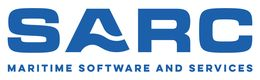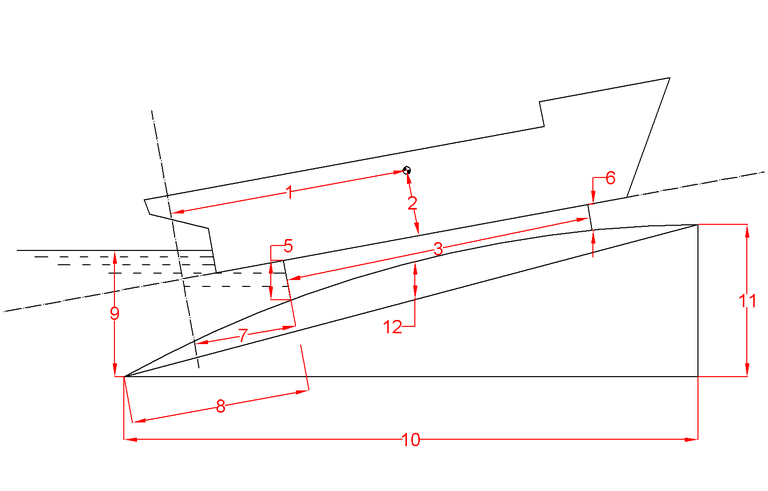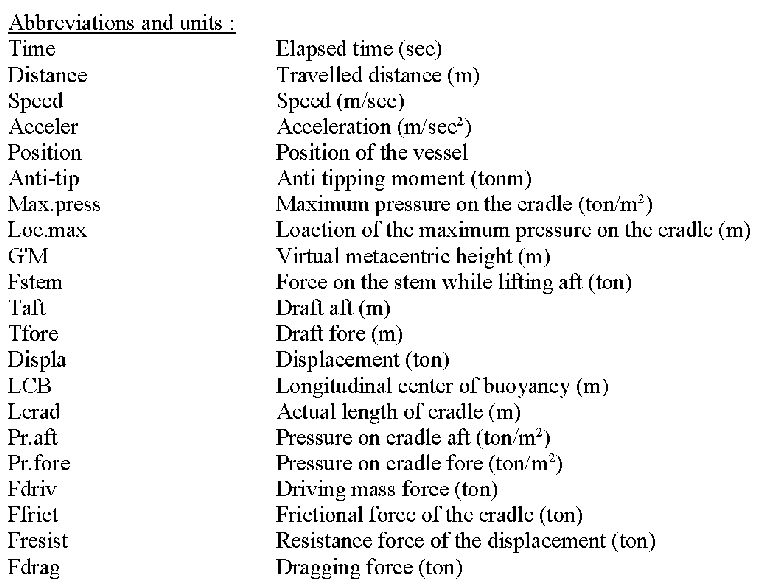PIAS Manual  2021 Program for the Integral Approach of Shipdesign
Launch: launching calculation
This module computes pressures, forces, speeds, anti-tipping moments etc. during longitudinal launching.

# Input data of ship and slipway

For ship and slipway, the following parameters — which are also illustrated in the sketch at the end of this section — must be provided.

• Identificatie, which is simply a name to be included in the output.
• The weight of the ship, during launching [ton].
• Longitudinal centre of gravity of the vessel is measured in the plane of the vessel in relation to APP.
• Vertical centre of gravity of the vessel is measured in the plane of the vessel in relation to the baseline.
• Length of fore poppet in relation with length of cradle [%], is that part of the cradle that is supposed to give upward force after floating of the aftship.
• Cradle height aft and for is measured perpendicularly to the cradle.
• Distance of aftside of the cradle to APP, and to the end of the slipway are measured alongside the slipway.
• Water level above the end of the way is measured perpendicularly to the water level.
• Density of outside water, which will (only for Launch) overwrite the density setting of Config — in ‘Calculation methods and output preference’, Density outside water.
• Camber of the slipway is measured at half-length of the way, perpendicularly to the slipway.
• Time interval for calculation, should be set to approximately 0.5 to 2 seconds in order to get an accurate calculation.
• Margin speed at which the calculation ends, has to be set because the calculation will otherwise continue endlessly when no dragging forces have been specified. The reason for this is that the water resistance will approximate to zero but will never become zero.

This parameter menu has an addition function [PAper size] which can be used to toggle between A3 and A4 paper size for formatting the output.Geometric parameters, with the numbers referring to the table below.
 1 Longitudinal center of gravity 2 Vertical center of gravity 3 Length of the cradle 5 Crade height aft 6 Crade height forward 7 Distance from aftside of the cradle to APP 8 Distance from the aftside of the cradle to aftside of the slipway 9 Waterlevel above the aftside of the slipway 10 Length of the slipway 11 Height at the forward side of the slipway 12 Camber at half length of the slipway

# Input data of the friction coefficient of the cradle

One or more dimensionless friction coefficients as a function of the travelled distance are defined in this input screen, as illustrated in the figure below. The friction coefficient is defined as ship weight / friction force, both in the same dimension. The friction coefficient is assumed to be independant of speed. In this input menu a number (maximum 40) of travelled distance & friction coefficient combinations can be defined. Intermediate coefficients are calculated by linear interpolation, with at the extremities the provisions as illustrated in the figure below:

• At a travelled distance less that the value of the first row, the friction coefficient will be taken as zero.
• At travelled distances larger than the last row, the friction coefficient from the last row will be taken.Ttravelled distance & friction coefficient.

# Input data of the resistance coefficient of the wetted hull

One or more resistance coefficients of the wetted hull can be defined here as a function of the speed. This coefficient is defined in its simplest form as follows: coefficient = resistance / displacement / speed2 [sec2/m2]. For interpolation of intermediate values the same procedure is adopted as described above.

# Input data of the dragging forces

One or more dragging forces can be defined here as a function of the speed. Intermediate values are calculated as described above.

# Execute launching calculation

For every step in time the speed, pressure, forces, and travelled distance is printed, as in the example below. By the way, the assumption in the cradle pressure is that it is linearly distributed over the cradle length, and that it is only positive (after all the cradle cannot exert a tension force).Example of a single page of output.Explanation of the output parameters.

# File and backup management

Backups can be made and restored here. Here is also the option ‘Quit module without saving the data’. For details we refer to Data storage and backups.